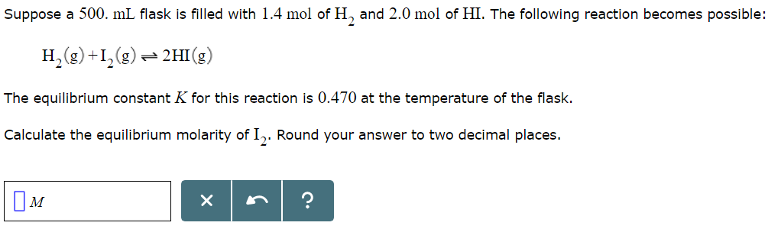# Suppose a 500. mL flask is filled with 1.4 mol of H2 and 2.0 mol of HI. The following reaction becomes possible: H2(g) + I2(g) ⇌ 2HI(g) The equilibrium constant K for this reaction is 0.470 at the temperature of the flask. Calculate the equilibrium molarity of I2. Round your answer to two decimal places.